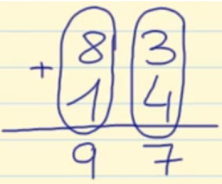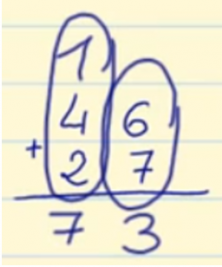Smartick is an advanced online program that teaches kids math and coding in only 15 min. a day
Try it for free!

Jul06

# How to Add with and without Regrouping

In this post, we are going to learn how to do addition problems with and without regrouping.

### How to Add without Regrouping

• Place the addends one on top of the other so that the place values fall in the same columns.
• Add each column together separately, starting with the 1s place.
• The sums go below each column, underneath the line.

Let’s take a look at an example adding 83 + 14.

We start by writing the number 83 and below it, the number 14, making sure to line up the 1s columns, or in other words, the 3 and 4.

We then add 3 + 4 = 7 and write it underneath the 1s place column. Then, we add 8 + 1 = 9 and we write it under the 10s place column.And we get the answer 97.

### How to Add with Regrouping

If we get a two digit number while adding the first column together, we add that number to the 10s place column.

Let’s check out an example adding 46 + 27.

First, we write the 46 underneath, making sure to line up the 1s place columns and lastly, we write down the 27.

We first need to add the 1s place numbers 6 + 7 = 13, we need to put the 3 underneath the 1s place column and add the 1 to the 10s place column.

1 + 4 + 2 = 7, we write the 7 underneath in the 10s place column.And the answer of the addition problem is 73.

If you want to practice these kinds of problems, and more, go to Smartick and try it for free!

Learn More:

Latest posts by Smartick (see all)